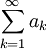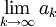# Back to The Divergence Test

Previous: Contrapositive Example with Sets

Next: Proof of the Ratio Test

## Back to the Divergence Test

The given statement

If the seriesis convergent, then the limitequals zero

is certainly true. We were able to prove it in our lesson on the Divergence Test. We can construct the contrapositive of this statement by reversing the conditions and making them false:

If the limitis not zero, then the seriesis not convergent.

The contrapositive statement is the Divergence Test.

Now, we know that the contrapositive of a given statement is true if the given statement is true.

But the original statement is true, so the contrapositive is also true. That is, the Divergence Test requires no proof, because it is the contrapositive of a statement that is true.